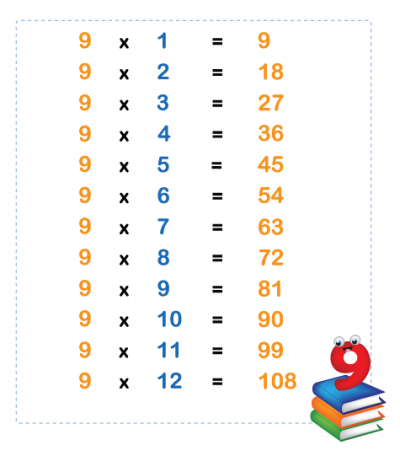The fun thing about learning the 9 times table is that there is a trick.

All the numbers in the 9 times table add up to 9! Have a look:9 x 2 = 18      1 + 8 = 9
9 x 3 = 27      2 + 7 = 9
9 x 4 = 36      3 + 6 = 9